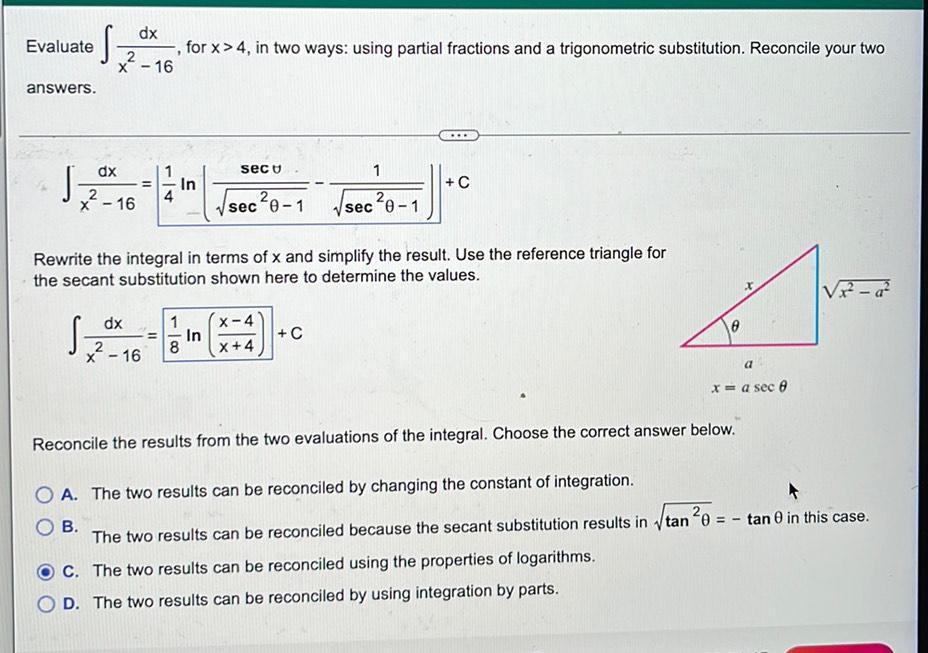Question:

# Evaluate dx 2 x - 16 for x > 4, in two ways: using partial fractions and a trigonometric substitution. Reconcile your two - answEvaluate dx 2 x - 16 for x > 4, in two ways: using partial fractions and a trigonometric substitution. Reconcile your two - answers. ... dx secu 1 J 2 sec?0-1 Vsec²0-1 II In +C 2 x - 16 4 Rewrite the integral in terms of x and simplify the result. Use the reference triangle for the secant substitution shown here to determine the values. Vir - 22 Sza dx 1 = In 8 x - 16 X-4 X+4 +C a x = a seco Reconcile the results from the two evaluations of the integral. Choose the correct answer below. O A. The two results can be reconciled by changing the constant of integration. OB The two results can be reconciled because the secant substitution results in itan ²0 = - tan 0 in this case. C. The two results can be reconciled using the properties of logarithms. D. The two results can be reconciled by using integration by parts.